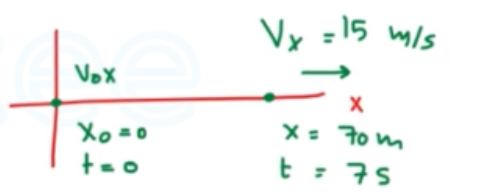Need Help?

Subscribe to Physics 1

###### \${selected_topic_name}
• Notes
• Comments & Questions

$\begin{array}{l}{\text { An antelope moving with constant acceleration covers the distance between two }} \\ {\text { points } 70.0 \mathrm{m} \text { apart in } 7.00 \mathrm{s} \text { . Its speed as it passes the second point is } 15 \mathrm{m} / \mathrm{s}} \\ {\text { (a) What is its speed at the first point? (b) What is its acceleration? }}\end{array}$$x-x_{0}=70 \mathrm{m}$

$t=7 s$

$V_{x}=15 \mathrm{m} / \mathrm{s}$

$V_{0 x}=? ?$

$x-x_{0}=\left(\frac{V_{0 x}+V_{x}}{2}\right) t$

$\rightarrow V_{0} x=\frac{2\left(x-x_{0}\right)}{t}-V_{x}$

$=\frac{2(70 \mathrm{m})}{7 \mathrm{s}}-15 \mathrm{m} / \mathrm{s}$

$=5 \mathrm{m} / \mathrm{s}$

$V_{x}=V_{0 x}+a_{x} t$

$\rightarrow a_{x}=\frac{v_{x}-v_{0x}}{t}=\frac{15-5}{7}$

$=1.43 \mathrm{m} / \mathrm{s}^{2}$

$\begin{array}{l}{\text { The fastest measured pitched baseball left the pitcher's hand at a speed of } 45 \mathrm{m} / \mathrm{s} \text { if the pitcher was in }} \\ {\text { contact with the ball over a distance of } 1.50 \mathrm{m} \text { and produced constant acceleration, (a) what }} \\ {\text { acceleration did he give the ball, and (b) how much time did it take him to pitch it? }}\end{array}$

$x-x_{0}=1.5 \mathrm{m}, \quad \mathrm{V}_{x}=45 \mathrm{m} / \mathrm{s} \quad, \quad \mathrm{V}_{0}=0$

$V_{x}^{2}=V_{0 x}^{2}+2 a_{x}\left(x-x_{0}\right)$

$\rightarrow a_{x}=\frac{v_ x^{2}-v_{0 x}^{2}}{2\left(x-x_{0}\right)}=\frac{\left(45\right)^{2}}{2(1.5)}=675 \mathrm{m} / \mathrm{s}^{2}$

$x-x_{0}=\left(\frac{V_{0 x}+V_{x}}{2}\right) t \rightarrow t=\frac{2\left(x-x_{0}\right)}{V_{0 x}+V_{x}}$

$=\frac{2(1.5)}{45}=0.0667 \mathrm{s}$

OR

$V_{x}=V_{0 x}+a_{x} t \rightarrow t=\frac{V_{x}}{a_{x}}=\frac{45}{675}=0.0667 \mathrm{s}$

$\begin{array}{l}{\text { A Tennis Serve. In the fastest measured tennis serve, the ball left the racquet at } 73.14 \mathrm{m} / \mathrm{s} \text { . A served tennis ball is typically }} \\ {\text { in contact with the racquet for } 30.0 \mathrm{ms} \text { and starts from rest. Assume constant acceleration. (a) What was the ball's }} \\ {\text { acceleration during this serve? (b) How far did the ball travel during the serve? }}\end{array}$

$V_{x}=73.14 \mathrm{m/s}, \quad \mathrm{V}_{0 x}=0 \quad \text { and } \quad t=30 \mathrm{ms}$

$V_{x}=V_{0 x}+a_{x} t \rightarrow a_{x}=\frac{V_{x}-V_{0 x}}{t}$

$∴ a_{x}=\frac{73.14-0}{30 * 10^{-3}}=2440 \mathrm{m} / \mathrm{s}^{2}$

$x-x_{0}=\left(\frac{V_{0 x}+V_{x}}{2}\right) t=\left(\frac{0+73.14}{2}\right)\left(30* 10^{-3}\right)=1.1 \mathrm{m}$

OR

$x-x_{0}=v _{0 x}t+\frac{1}{2} a_{x} t^{2}=\frac{1}{2}(2440)\left(30*10^{-3}\right)^{2}$

$=1.1 \mathrm{m}$

No comments yet